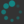1. Consider a regular $n$-gon inscribed in a circle of radius 1: Show that its perimeter is given by $2n \sin\left(\frac{360^\circ}{2n}\right).$
2. Recall that $$\Twopi$$ is defined to be the circumference of a unit circle, and $\pi$ is defined to be half this quantity. Compute approximate values of $$\Twopi$$ and $\pi$ (to 4 decimal points, say).